10/22/19 at 02:56:40 News: Registration for new users has been disabled to discourage spam. If you would like to join the forum please send me an email with your desired screen name to tony at gravitysimulator dot com.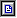Pages: 1 2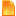faster and better method of integration (Read 15806 times)
 wil UploaderI Love YaBB 2! Posts: 41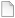faster and better method of integration 05/22/15 at 14:39:23   If the Gravity Simulator is still alive, then I can send my code of the new method of integration.   The method is quite new, probably still unknown yet. It's a generalised version of the Sturmer-Nystrom methods, based on an extrapolation.   I made my algorithm (coded in MS C++) basing on this work: http://arxiv.org/pdf/0809.0914.pdf   The code is very simple, probably even simpler than the RK4 methot, but haigly better, because the order of the integrator can be arbitrality: 2, 4, 6, 8, ... up to 16 or 20 for a double precision computiotions.   It's very fast and precise... probably about 100 times faster then the RK4, still for the 8 order settings.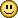Back to top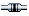IP Logged
 Tony YaBB Administrator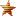Posts: 1060 Gender: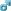Re: faster and better method of integration Reply #1 - 05/22/15 at 23:07:40   Sure, I'd love to see your code.  If I can feed it the x,y,z's and vx, vy, and vz's of every object in a system, and it can feed me back the new values after an iteration, it should be able to plug in just fine. Back to topIP Logged
 wil UploaderI Love YaBB 2! Posts: 41Re: faster and better method of integration Reply #2 - 05/23/15 at 06:36:08   Of course, it's possible...   Actualy I used the gaussian units: k - the gauss constant (instead of the G), 1au - a distance uinit, and 1 day - a time unit.   const double au = 149597870.700; // km const double k_g = 0.01720209895; //     Fourther I multiply any mass by k^2, because it simplifies the forces calculation, which looks like this:   void force(TP3d *o, TBody *y, int n) {   TBody *e = y + n-1;         zero(o); // the table of bodie's accelerations       for(TBody *p = y; p < e; p++, o++)   {       TBody *q = p; TP3d *a = o;       do{      q++; a++;        TP4d r; r.sub(p->r, q->r);              r.s = r*r; r.s = 1.0 / (r.s*sqrt(r.s));        o->subm(r, q->m*r.s);          a->addm(r, p->m*r.s);           }while( q < e );   } }   Where the TBody is a structure of the data:   double m;         // a mass of body multiplied by k^2 double nothing; // alignment... to be 64 bytes of the whole size TP3d r, v;         // a position and velocity vectors: x,y,z Back to topIP Logged
 Tony YaBB AdministratorPosts: 1060 Gender:Re: faster and better method of integration Reply #3 - 05/23/15 at 13:03:22   Hi Wil, I'm not very familiar with C++, so its difficult for me to follow your code.  Would you know how to write it in Javascript?   Your function would look like this: Code:function SturmerNystrom() {   for (iteration = 1; iteration <= iterationsPerVisit; iteration++) {   // your integration code here   } }   This function assumes the following global variables exist:   iterationsPerVisit - tells the function how many iterations to perform.  High values mean fast simulations at the expense of jumpy graphics.  iterationsPerVisit = 100 is what I usually use. objects - the number of objects in the simulation objMass[n] - the mass of each object in the simulation objx[n] - array of objects containing the x position of each object in the simulation objy[n] - array of objects containing the y position of each object in the simulation objz[n] - array of objects containing the z positionof each object in the simulation objvx[n] - array of objects containing the x velocitiy of each object in the simulation objvy[n] - array of objects containing the y velocitiy of each object in the simulation objvz[n] - array of objects containing the z velocitiy of each object in the simulation G - Universal Grivitational Constant.  I like to express mass in Earth's mass, so G = 398600432896939 N m^2 / Mearth^2 rather than the more familiar G = 6.67e-11 N m^2 / kg^2; timeStep - the amount of time (seconds) to integrate in each iteration.   If you can adapt your code to this format, I can try it.   In the next post in this thread, I'll show you Gravity Simulator's RK4() javascript integrator. Back to topIP Logged
 Tony YaBB AdministratorPosts: 1060 Gender:Re: faster and better method of integration Reply #4 - 05/23/15 at 13:05:27   continued from previous post   Here's Gravity Simulator's javascript version of the RK4 function. Code:function RK4() {      for (iteration = 1; iteration <= iterationsPerVisit; iteration++) {         //=======================  RK4 ==========================================         //Use current positions to compute accelerations.  Store them in arrays a1x[], a1y[], a1z[].         //zero out arrays a1x[], a1y[], a1z[]         for (k = 1; k <= objects; k++) {       a1x[k] = 0; a1y[k] = 0; a1z[k] = 0;         } // Next k         for (k = 1; k <= objects; k++) {       Mu = objMass[k] * G;            for (j = k + 1; j <= objects; j++) {               Mu2 = objMass[j] * G;               dx = (objx[j] - objx[k]);               dy = (objy[j] - objy[k]);               dz = (objz[j] - objz[k]);               D = Math.sqrt(dx * dx + dy * dy + dz * dz);               if (Mu > 0) { //ignore massless particles                  a1 = -(1 / D) * (1 / D) * Mu;                  a1x[j] = a1x[j] + (dx / D) * a1;                  a1y[j] = a1y[j] + (dy / D) * a1;                  a1z[j] = a1z[j] + (dz / D) * a1;                } // End if                if (Mu2 > 0) { //ignore massless particles                   a1 = -(1 / D) * (1 / D) * Mu2;                   a1x[k] = a1x[k] - (dx / D) * a1;                   a1y[k] = a1y[k] - (dy / D) * a1;                   a1z[k] = a1z[k] - (dz / D) * a1;                } // End if            } // Next j         } // Next k         //Copy the current velocities into an arrays v1x[], v1y[], v1z[].         for (k = 1; k <= objects; k++) {            v1x[k] = objvx[k];            v1y[k] = objvy[k];            v1z[k] = objvz[k];         }         //Use the velocities stored in v1x[], v1y[], v1z[] to predict positions of objects one-half a step into the future.         //Store the positions in arrays p2x[], p2y[], p2z[]         halfstep = timeStep / 2;         for (k = 1; k <= objects; k++) {            p2x[k] = objx[k] + v1x[k] * halfstep;            p2y[k] = objy[k] + v1y[k] * halfstep;            p2z[k] = objz[k] + v1z[k] * halfstep;         } // Next k           //Use the accelerations stored in a1x[], a1y[], a1z[] to predict future velocities at half a step into the future.         //Store the velocities in arrays v2x[], v2y[], v2z[].         for (k = 1; k <= objects; k++) {            v2x[k] = objvx[k] + a1x[k] * halfstep;            v2y[k] = objvy[k] + a1y[k] * halfstep;            v2z[k] = objvz[k] + a1z[k] * halfstep;         } // Next k         //Use the positions stored in arrays p2x[], p2y[], p2z[] (these are the positions at half a step in future)         //to compute accelerations on the bodies a half timestep into the future.         //Store these accelerations in arrays a2x[], a2y[], a2z[]         //zero out arrays a2x[], a2y[], a2z[]         for (k = 1; k <= objects; k++) {            a2x[k] = 0; a2y[k] = 0; a2z[k] = 0;         }         for (k = 1; k <= objects; k++) {            Mu = objMass[k] * G;            for (j = k + 1; j <= objects; j++) {               Mu2 = objMass[j] * G;               dx = (p2x[j] - p2x[k]);               dy = (p2y[j] - p2y[k]);               dz = (p2z[j] - p2z[k]);               D = Math.sqrt(dx * dx + dy * dy + dz * dz);               if (Mu > 0) { //ignore massless particles                  a2 = -(1 / D) * (1 / D) * Mu;                  a2x[j] = a2x[j] + (dx / D) * a2;                  a2y[j] = a2y[j] + (dy / D) * a2;                  a2z[j] = a2z[j] + (dz / D) * a2;               } // End if               if (Mu2 > 0) { //ignore massless particles                  a2 = -(1 / D) * (1 / D) * Mu2;                  a2x[k] = a2x[k] - (dx / D) * a2;                  a2y[k] = a2y[k] - (dy / D) * a2;                  a2z[k] = a2z[k] - (dz / D) * a2;               } // End if            } // Next j         }  // Next k           //Use the accelerations stored in arrays a2x[], a2y[], a2z[] to compute velocities at half a step into the future.         //Store these velocities in arrays v3x[], v3y[], v3z[].         for (k = 1; k <= objects; k++) {            v3x[k] = objvx[k] + a2x[k] * halfstep;            v3y[k] = objvy[k] + a2y[k] * halfstep;            v3z[k] = objvz[k] + a2z[k] * halfstep;         } // Next k         //Use the velocities stored in arrays v2x[], v2y[], v2z[]         //to predict positions of objects one-half a step into the future.         //Store these positions in arrays p3x[], p3y[], p3z[].         for (k = 1; k <= objects; k++) {            p3x[k] = objx[k] + v2x[k] * halfstep;            p3y[k] = objy[k] + v2y[k] * halfstep;            p3z[k] = objz[k] + v2z[k] * halfstep;         } // Next k                 //Use the positions in arrays p3x[], p3y[], p3z[] (these positions are at half a step in future)         //to compute the accelerations on the bodies a half time step into the future.         //Store them in arrays a3x[], a3y[], a3z[]         for (k = 1; k <= objects; k++) {            a3x[k] = 0; a3y[k] = 0; a3z[k] = 0;         }         for (k = 1; k <= objects; k++) {            Mu = objMass[k] * G;            for (j = k + 1; j <= objects; j++) {               Mu2 = objMass[j] * G;               dx = (p3x[j] - p3x[k]);               dy = (p3y[j] - p3y[k]);               dz = (p3z[j] - p3z[k]);               D = Math.sqrt(dx * dx + dy * dy + dz * dz);               if (Mu > 0) { //ignore massless particles                  a3 = -(1 / D) * (1 / D) * Mu;                  a3x[j] = a3x[j] + (dx / D) * a3;                  a3y[j] = a3y[j] + (dy / D) * a3;                  a3z[j] = a3z[j] + (dz / D) * a3;               } // End if               if (Mu2 > 0) { //ignore massless particles                  a3 = -(1 / D) * (1 / D) * Mu2;                  a3x[k] = a3x[k] - (dx / D) * a3;                  a3y[k] = a3y[k] - (dy / D) * a3;                  a3z[k] = a3z[k] - (dz / D) * a3;               } // End if            }  // Next j         } // Next k         //Use the accelerations in arrays a3x[], a3y[], a3z[] to compute velocities at one FULL step into the future.         //Store them in arrays v4x[], v4y[], v4z[].         for (k = 1; k <= objects; k++) {            v4x[k] = objvx[k] + a3x[k] * timeStep;            v4y[k] = objvy[k] + a3y[k] * timeStep;            v4z[k] = objvz[k] + a3z[k] * timeStep;         } // Next k         //Use the veolcities stored in arrays v3x[], v3y[], v3z[]         //to compute the positions of objects one FULL step into the future.         //Store them in arrays p4x[], p4y[], p4z[].         for (k = 1; k <= objects; k++) {            p4x[k] = objx[k] + v3x[k] * timeStep;            p4y[k] = objy[k] + v3y[k] * timeStep;            p4z[k] = objz[k] + v3z[k] * timeStep;         } // Next k         //Use the positions stored in arrays p4x[], p4y[], p4z[](these are the positions at one FULL step in future)         //to compute accelerations on the bodies in the future.         //Store these accelerations in arrays a4x[], a4y[], a4z[]         for (k = 1; k <= objects; k++) {            a4x[k] = 0; a4y[k] = 0; a4z[k] = 0;         } // Next k continued on next page due to 10000 char limit per post. Back to topIP Logged
 Tony YaBB AdministratorPosts: 1060 Gender:Re: faster and better method of integration Reply #5 - 05/23/15 at 13:07:00   continued from previous post Code:         for (k = 1; k <= objects; k++) {            Mu = objMass[k] * G;            for (j = k + 1; j <= objects; j++) {               Mu2 = objMass[j] * G;               dx = (p4x[j] - p4x[k]);               dy = (p4y[j] - p4y[k]);               dz = (p4z[j] - p4z[k]);               D = Math.sqrt(dx * dx + dy * dy + dz * dz);               if (Mu > 0) { //ignore massless particles                  a4 = -(1 / D) * (1 / D) * Mu;                  a4x[j] = a4x[j] + (dx / D) * a4;                  a4y[j] = a4y[j] + (dy / D) * a4;                  a4z[j] = a4z[j] + (dz / D) * a4;               } // End if               if (Mu2 > 0) { //ignore massless particles                  a4 = -(1 / D) * (1 / D) * Mu2;                  a4x[k] = a4x[k] - (dx / D) * a4;                  a4y[k] = a4y[k] - (dy / D) * a4;                  a4z[k] = a4z[k] - (dz / D) * a4;               } // End if            } // Next j         } // Next k         //Apply the Runge-Kutta 4th Order method to these arrays to determine the slope of the position and velocities         //Then add these slopes to the current positions and velocities to get the new positions and velocities.         //Velocity is the derivative of position.         //Acceleration is the derivative of velocity.         //The RK4 method states that the slope for velocity is         // (1/6) * timeStep * (the acceleration at the beginning of the interval, stored in a1x[], a1y[], a1z[]         // + 2 * the acceleration at the first estimate of the half time step, stored in a2x[], a2y[], a2z[]         // + 2 * the acceleration at the second estimate of the half time step, stored in a3x[], a3y[], a3z[]         // + the acceleration at the estimate of the end of a full time step, stored in a4x[], a4y[], a4z[] ).         //The RK4 method states that the slope for position is         // (1/6) * timeStep * (the velocity at the beginning of the interval, stored in v1x[], v1y[], v1z[]         // + 2 * the velocity at the first estimate of the half time step, stored in v2x[], v2y[], v2z[]         // + 2 * the velocity at the second estimate of the half time step, stored in v3x[], v3y[], v3z[]         // + the velocity at the estimate of the end of a full time step, stored in v4x[], v4y[], v4z[] ).         for (k = 1; k <= objects; k++) {            deltavx = (timeStep / 6) * (a1x[k] + 2 * a2x[k] + 2 * a3x[k] + a4x[k]);            deltavy = (timeStep / 6) * (a1y[k] + 2 * a2y[k] + 2 * a3y[k] + a4y[k]);            deltavz = (timeStep / 6) * (a1z[k] + 2 * a2z[k] + 2 * a3z[k] + a4z[k]);              objvx[k] = objvx[k] + deltavx;            objvy[k] = objvy[k] + deltavy;            objvz[k] = objvz[k] + deltavz;         } // Next k         iterations++;     } // Next iteration   } // End function rk4 Back to top « Last Edit: 05/23/15 at 20:32:05 by Tony »IP Logged
 frankuitaalst Ultimate Member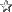Great site Posts: 1510 Gender:Re: faster and better method of integration Reply #6 - 05/24/15 at 04:53:52   Quote from wil on 05/22/15 at 14:39:23:If the Gravity Simulator is still alive, then I can send my code of the new method of integration. The method is quite new, probably still unknown yet. It's a generalised version of the Sturmer-Nystrom methods, based on an extrapolation. I made my algorithm (coded in MS C++) basing on this work: http://arxiv.org/pdf/0809.0914.pdf The code is very simple, probably even simpler than the RK4 methot, but haigly better, because the order of the integrator can be arbitrality: 2, 4, 6, 8, ... up to 16 or 20 for a double precision computiotions. It's very fast and precise... probably about 100 times faster then the RK4, still for the 8 order settings.This is great news !   Have you been able to do some simulations in order to check speed and accurancy ?   I mean : introducing higher order in the integration may allow to set larger timesteps for a given "accurancy" . A simple test may be performed : set up a system with central mass and a test particle .   The particle should stay within ...x AU after  T time at a timestep of dt .   Anxious to see how the integrator performs in GravSim ... I personally use sometimes a 21-order Taylor integrator when I want accurate simulations with self adapting time step . Back to top « Last Edit: 05/24/15 at 08:22:56 by frankuitaalst »IP Logged
 wil UploaderI Love YaBB 2! Posts: 41Re: faster and better method of integration Reply #7 - 05/24/15 at 17:25:00   Quote from Tony on 05/23/15 at 13:03:22:Hi Wil, I'm not very familiar with C++, so its difficult for me to follow your code.  Would you know how to write it in Javascript? Your function would look like this: Code:function SturmerNystrom() {   for (iteration = 1; iteration <= iterationsPerVisit; iteration++) {   // your integration code here   } } This function assumes the following global variables exist: iterationsPerVisit - tells the function how many iterations to perform.  High values mean fast simulations at the expense of jumpy graphics.  iterationsPerVisit = 100 is what I usually use. objects - the number of objects in the simulation objMass[n] - the mass of each object in the simulation objx[n] - array of objects containing the x position of each object in the simulation objy[n] - array of objects containing the y position of each object in the simulation objz[n] - array of objects containing the z positionof each object in the simulation objvx[n] - array of objects containing the x velocitiy of each object in the simulation objvy[n] - array of objects containing the y velocitiy of each object in the simulation objvz[n] - array of objects containing the z velocitiy of each object in the simulation G - Universal Grivitational Constant.  I like to express mass in Earth's mass, so G = 398600432896939 N m^2 / Mearth^2 rather than the more familiar G = 6.67e-11 N m^2 / kg^2; timeStep - the amount of time (seconds) to integrate in each iteration. If you can adapt your code to this format, I can try it. In the next post in this thread, I'll show you Gravity Simulator's RK4() javascript integrator.   My code is very simple, because the extrapolation is based on a 2-order method - trapesoidal (thus a T symbol in the paper).   Maybe some theoretical intro...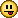The 2-order method is just the standard Velocity Verlet and LeapFrog methods. These are just a two variants... called as: VV and PV (P-position, V-velocity). And it's all, what we need.   VV:   v += a(r)*h/2; // a(r) has been computed earlier r += v*h;        // after this we must compute new: a(r) = f(r), because the position - r changes v += a(r)*h/2; //   PV: r += v(r)*h/2; // then we must compute: a(r) = f(r) v += a*h;      // r += v(r)*h/2; //   [ x += y is simply an increment the x by y: x = x + y;]   We can use any of these two versions of the 2-order mehods, several times, with a different time step, then combine the all results... and this is just called as an extrapolation.   The 4-order method looks just that: -1/3 T(h) + 4/3 T(h/2);   so, we must compute two times the same (final position): one with a step h, which gives some result: T(h) and secondly with a step: h/2, which gives the result: T(h/2).   Now we just must combine the both, to get the better result - correct up to 4-orger of the step h.   8-order method: 1/360 T(h) - 16/45 T(h/2) + 729/280 T(h/3) - 1024/218 T(h/4)   so, we just use 4 results fot the same final point, with a steps: h, h/2, h/3, h/4, and combine these... appropiately, to cancel the errors of h^2 up to h^8, then we get the result with error of order h^9 only, thus the method is 8-order. .... Back to topIP Logged
 wil UploaderI Love YaBB 2! Posts: 41Re: faster and better method of integration Reply #8 - 05/24/15 at 18:43:16   OK. This is a code of the method.     void stepk(int k) // order is 2k, then for k = 1 this is just a standard verlet. {     if( lastk != k ) calcc(k); // calculates the coeficients     force(a, y, nB); // only for VV - verlet, because the frog do not use the accel at a start point     TBody *ys = y+nB, *yi = ys+nB; // we need memory for two temporaliry tables: r, v of the bodies     h = dt; // dt is a global time step     copy(ys, y); // ys = y;  y is a table with r0 and v0 - initial point (previous position)     verlet(a, ys, 1); // one step only     for(int i = 2; i <= k; i++)    {      h = dt * i_m[i]; // h = 1/2, 1/3, ...          copy(yi, y); // yi = y,  copies of r and v for every body to yi (a table of objects)        verlet(a, yi, i); // computes new position on yi, with i steps... starting on yi, result is stored to yi also (r and v for all bodies).          add_y(ys,yi, nB, c_i, i); // extrapolation - combines two results of the tables: ys and yi; the result is stored to ys.    }      copy(y,ys);  // final result is in ys, and now copied to the y. }     And the calculation of these coefficients in the extrapolation:   void calck(double *c, int k) {   lastk = k;         c = 0;   for(int i = 1; i <= k; i++)    {      double m, l; m = l = 1.0; // double instead of int, due to overflow possib.        for(int j = 1; j <= k; j++)      if( i != j )         {           l *= i*i;             m *= i*i - j*j;         }                   c[i] = l / m;       c += c[i];         i_m[i] = 1.0 / i;   // 1, 1/2, 1/3, 1/4 ...        } } Back to topIP Logged
 wil UploaderI Love YaBB 2! Posts: 41Re: faster and better method of integration Reply #9 - 05/24/15 at 19:41:43   Quote from frankuitaalst on 05/24/15 at 04:53:52: Have you been able to do some simulations in order to check speed and accurancy ? I mean : introducing higher order in the integration may allow to set larger timesteps for a given "accurancy" .   Yes. I tested this... but not too much... because it's rather hard to test of methods of order over... 4 even.   A speed is very good, about 100 years/s for 8-order, for 16 it is 1/3 less only, for the 11 bodies: planets + Sun + Moon.   The author itself compares this method with many other, and it's looks the best - an optimal in a sense of the precision and a speed also, excluding some degenerate cases, which are possible but only for lower order... below 8 probably.I tested it also on the simple two body problem with extreme eccentricity: e = 0.999, and with a fixed step (a version with an adaptive step is rather simple to implement to this method...).   For the low order integration the system is very unstable... only for 12, or more, it keeps good. I use usually dt = 1/64 of a day = 22.5 minutes for a simulation of the Moon orbit... even for 4 order method it looks good.   For order 16 it's possible: h = 1 day, or 1/2 at least... but it is rather hard to test.   Higher order of integrator, ie. over 20, is rather useless with a double precision... for a quadruple precision (~100 bits) is probably a limit to 50. Back to topIP Logged
 wil UploaderI Love YaBB 2! Posts: 41Re: faster and better method of integration Reply #10 - 05/24/15 at 20:17:17   function SturmerNystrom() {    for (iteration = 1; iteration <= iterationsPerVisit; iteration++) {      // your integration code here       stepk(k); // order = 2k, k = 1, 2, ... 10.      } }Back to topIP Logged
 Tony YaBB AdministratorPosts: 1060 Gender:Re: faster and better method of integration Reply #11 - 05/25/15 at 09:54:48   Hi Wil.  Reading someone else's code is always a chore, especially if it in a language I'm not very familiar with.  I have a few questions.   If I understand this code, y is a table that contains the starting position and velocity vectors? Visual Basic and javascript can't do math functions on entire tables (or at least I don't know how), so I need to decompose these into their components: objx[], objy[], objz[], and objvx[], objvy[], objvz[].     Code:if( lastk != k ) calcc(k); // calculates the coeficients Where is the code for calcc(k)?   Code:void calck(double *c, int k) Is this it? Did you mean to call this calcc instead of calck?  If so, why does the above call pass only one parameter (k), while the function expects 2 (c, k)?   Code:force(a, y, nB); // only for VV - verlet, because the frog do not use the accel at a start point I imagine this function computes the net force on each object in the system: F=GMm/d^2.  What do "a" and "nB" represent? Is nB number of bodies?  If so, that's what I call "objects" in the javascript code.   Code:TBody *ys = y+nB, *yi = ys+nB; // we need memory for two temporaliry tables: r, v of the bodies This is where I need your help.  I'm not very familiar with C++.  I see this as Tbody multiplied by ys = y + nB, and nothing multiplied by yi = ys + nB.  I imagine the * has to do with variable declaration rather than multiplication?   Code:      h = dt * i_m[i]; // h = 1/2, 1/3, ... i_m is an array created in calck that contains 1, 1/2, 1/3, 1/4...? Did we need to do h = dt 4 lines up, since h is is being overwritten here before it was ever used?   Code:        verlet(a, yi, i); // computes new position on yi, with i steps... starting on yi, result is stored to yi also (r and v for all bodies). I have a Verlet routine for Gravity Simulator that I can use.  But what am I feeding it?  What is "a"?  What is "i"?  Is this the same "i" used as a loop counter in calck()?  If so, it should contain the value of k after exiting from that loop. Back to topIP Logged
 wil UploaderI Love YaBB 2! Posts: 41Re: faster and better method of integration Reply #12 - 05/25/15 at 14:01:27   I use structures or classes/objects for a data, therefore there are many arrays of objects, instead of simple vectors.   For exampe:   class TP3d {  public:   double x, y, z;   // and several simple procedures which operate on these x,y,z, for example: sum, sub, mul:    TP3d& add(TP3d &p) { x += p.x; y += p.y; z += p.z; return *this; }    void sub(TP3d &p) { ... }    mul(double m) { ... }; };   now we can compute in this way:   a.add(b);   what is a short version of: a.x = a.x + b.x; a.y = a.y + b.y; a.z = a.z + b.z;     ....   the calck is just calcc(c_i, k);  where c_i is an array of doubles.   force(a, y, nB); nB: number of bodies in the sim; a: it is an array of points: x,y,z, thus the TP3d class, of nB in size (at least); there the computed accelerations is stored y: an array of bodies     In C a pointer to an object, is formaly the same as an array of objects.   TP3d points; // array of 1000 points TP3d *a; // a is a pointer only...   a = points + 200; // now the pointer addreses these array, but at a position 200   a.x = 7; // in fact: points.x = 7   We can allocate dynamicaly also, of course - as in the java: a = new TP3d [n]; // n points in some ram... y = new TBody[3*n]; // room for 3 copies of n bodies: two additional copies for manipulation...   TBody *ys = y + nB; // ys points over the last body in y... first copy   // ys begins on the n-th body in thy y array; ys is the same place/data as y[n]   TBody *yi = ys + n; // and this points over the first copy - second copy .........   h = dt * i_m[i]; // h = 1/2, 1/3, ...     the h is also a global double, which keeps the actual time step, because it changes: h = dt, dt/2, dt/3, ... It can be simply:   h = dt/i;   but a divide operation is very slow.Back to topIP Logged
 wil UploaderI Love YaBB 2! Posts: 41Re: faster and better method of integration Reply #13 - 05/26/15 at 07:04:48   "Visual Basic and javascript can't do math functions on entire tables (or at least I don't know how), so I need to decompose these into their components: objx[], objy[], objz[], and objvx[], objvy[], objvz[]. "   Java is almost identical to C++; in the Java the points don't exist... there is just a reference. I don't know what are in the Basic, but I think there must be some reference or pointer also, because the addressing of a data is just... a basic operation in any programing.Show me for example a function in the Basic, which copies two arrays.   even in a pascal it is simple:   procedure copy(var a, b : array of some; n : integer); var i : integer; begin    for i := 0 to n-1 do    a[i] := b[i]; end;   Maybe I try to convert this to the Java... or Javascript?   I found in the Basic it's a 'ByRef' key, which is just a reference: https://msdn.microsoft.com/en-us/library/831f9wka.aspx Back to topIP Logged
 wil UploaderI Love YaBB 2! Posts: 41Re: faster and better method of integration Reply #14 - 05/28/15 at 10:59:51   Ok. Here You are the whole code in some simplified version, which can be understandable... easy modifable to any languige.   Code: // some global variables: double dt, h, h2; // time steps int nB; // number of bodies TP3d *a; // an array for accelerations of the bodies (any array must be created - a memory must be allocated, in some way... for the nB objects // and a table of objects: y, and two additional: ys, yi TBody *y, *ys, *y; // these keep: r, v vectors for any body, and a mass m // every of these structure can be replaced by six simple arrays of double, // therefore 6 arrays - because r and v, and plus one for m! // double objrx[],objry[],objrz[], objvx[],objvy[],objvz[]; // and the additional two: // double rxs[],ry[],rzs[], vxs[],vys[],vzs[]; // double rxi[],ryi[],rzi[], vxi[],vyi[],vzi[]; // and an rrray of masses of bodies, or rather a product: m = GM, for the bodies // double m[];   void force() {  zero(a); // a[...] = 0.0  for(int j = 0; j < nB-1; j++)  {    for(int i = j+1; i < nB; i++)    {      TP3d r = y[j].r - y[i].r; // r = rj - ri      double s = r*r; // s = |r|^2 = x^2 + y^2 + z^2;      s = 1.0 / (s*sqrt(s)); // s = 1/|r|^3      a[j] += r * y[i].m*s; // +G*mi/|r|^3 * r      a[i] -= r * y[j].m*s; // -G*mj/|r|^3 * r; rij == -rji    }  } } void frog(int k) { //      r += v(r)*h/2; // --> a(r) = f(r) //      v += a*h;      // //      r += v(r)*h/2; //     h2 = h*0.5;     do{      for(int i = 0; i < nB; i++)       {         y[i].r.addm(y[i].v, h2); // r += v*h2       }      force(); // a = f(r);      for(int i = 0; i < nB; i++)       {         y[i].v += a[i] * h;  // v += a*h         y[i].r += y[i].v * h2; // r += v*h2       }     }while( --k ); } //========== method order = 2k = 2 do 20; (k = 1..10) or more ... ============ double c_i[11+1], i_m[11+1]; int lastk = 0; void calcc(int k) {    lastk = k;  c_i = 1.0;  for(int i = 1; i <= k; i++)   {     double m, l; m = l = 1.0;     for(int j = 1; j <= k; j++)      if( i != j )       {         l *= i*i;         m *= i*i - j*j;       }     c_i[i] = l / m;     i_m[i] = 1.0 / i;   // 1, 1/2, 1/3, 1/4 ...   } } // k = 8 => n = 2k = 16; // c1 = 1/(1-2^2) * 1/(1-3^2) ... 1/(1-8^2) = -1/914457600 =~ -1e-9 // c8 = 64/(64-1) * ... 64/(64-49) = 4398046511104/163459296000 = 26.906... void addm(double m) {  for(int i = 0; i < nB; i++)  {    ys[i].r += y[i].r * m;    ys[i].v += y[i].v * m;  } } void stepk(int k) {  if( lastk != k ) calcc(k);  copy(y0, y); // copy y to y0  zero(ys);      for(int i = 1; i <= k; i++)   {      h = dt * i_m[i];      copy(y, y0);      frog(i); // i steps of a LeapFrog with a time setp = h = dt/i;      addm(c_i[i]);   }    copy(y,ys); }   Any operation on the r or v can be easily replaced to a three instructions on x,y and z, separately.     For example, the operation: ys[i].r += y[i].r * m;   should be replaced by: xs[i] += x[i] *m; ys[i] += y[i] *m; zs[i] += z[i] *m;   Additionaly the functions: force, frog, addm, ect, can be inlined directly in the place of a call... then only the one function leaves: stepk. proc.: copy (of array) also... Back to topIP LoggedPages: 1 2
 Forum Jump: ----------------------------- General -----------------------------=> Discussion